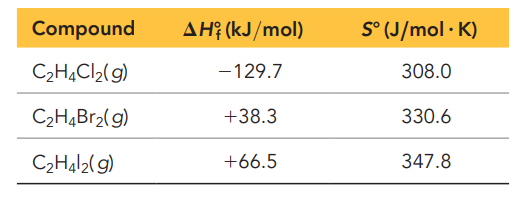×
Get Full Access to Chemistry: A Molecular Approach - 5 Edition - Chapter 19 - Problem 87
Get Full Access to Chemistry: A Molecular Approach - 5 Edition - Chapter 19 - Problem 87

×ISBN: 9780134874371 2047

## Solution for problem 87 Chapter 19

Chemistry: A Molecular Approach | 5th Edition

• Textbook Solutions
• 2901 Step-by-step solutions solved by professors and subject experts
• Get 24/7 help from StudySoup virtual teaching assistantsChemistry: A Molecular Approach | 5th Edition

4 5 1 326 Reviews
21
5
Problem 87

Ethene $$\left(\mathrm{C}_{2} \mathrm{H}_{4}\right)$$ can be halogenated by the reaction:

$$\mathrm{C}_{2} \mathrm{H}_{4}(g)+\mathrm{X}_{2}(g) \rightleftharpoons \mathrm{C}_{2} \mathrm{H}_{4} \mathrm{X}_{2}(g)$$

where $$\mathrm{X}_{2}$$ can be $$\mathrm{Cl}_{2}, \mathrm{Br}_{2}$$, or $$\mathrm{I}_{2}$$. Use the thermodynamic data given to calculate $$\Delta H^{\circ}, \Delta S^{\circ}, \Delta G^{\circ}$$, and $$K_{\mathrm{p}}$$ for the halogenation reaction by each of the three halogens at $$25^{\circ} \mathrm{C}$$. Which reaction is most spontaneous? Least spontaneous? What is the main factor responsible for the difference in the spontaneity of the three reactions? Does higher temperature make the reactions more spontaneous or less spontaneous?Text Transcription:

(C2H4)

C2H4( g) + X2( g) rightleftharpoons C2H4X2( g)

X_2

Cl_2, Br_2

I_2

Delta H^circ

Delta S^circ

Delta G^circ

K_p

25^circ C

Step-by-Step Solution:
Step 1 of 3

Name:__Rachel Jefferies__________________ 1.Stateandexplainthelawofconservationofmass The law of conservation of mass states that in a chemical reaction, matter is neither created or destroyed. So when combining two elements such as sodium and chloride, the mass of the compound is equal to the mass of both individual elements added together. This proves that no atoms were destroyed in the process. 2.Stateandexplainthelawofdefiniteproportions The law of definite proportions (or the law of constant composition) states that “All samples of a given compound, regardless of their source of how they were prepared, have the same proportions of their constituent elements”. Ex. Decomposition of 18 g of water = 16 g of oxygen and 2 g of hydrogen. Oxygen-Hydrogen Mass ratio = 16 g O/ 2 g H = 8.0 3.Stateandexplainthelawofmultipleproportions.Howisthelawofmultipleproportions different fromthelawofdefiniteproportions The law of multiple proportions states that, “When two elements form (call them A and B) two different compounds, the masses of element B that combines with 1 gram of element A can be expressed as a ratio of small whole numbers”. Ex. The mass ratio of oxygen to carbon in carbon dioxide is 2.67:1. Therefore, 2.67 g of oxygen reacts with 1 gram of carbon. In carbon monoxide, however, the mass ratio of oxygen to carbon is 1.33:1, or 1.33 g of oxygen to every 1 g of carbon. Mass oxygen to 1 g of carbon in carbon dioxide2.67 Mass oxygen to 1 g of carbon in carbon monox

Step 2 of 3

Step 3 of 3

## Discover and learn what students are asking

Statistics: Informed Decisions Using Data : Inference about the Difference between Two Medians: Dependent Samples
?In Problems 3–10, use the Wilcoxon matched-pairs signedranks test to test the given hypotheses at the a = 0.05 level of significance. The dependent sa

Unlock Textbook Solution